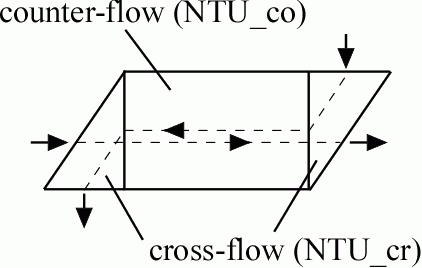Contents - IndexThe function provides the effectiveness of a counterflow heat exchanger that has cross-flow headers.  The solution is obtained using the method suggested by Kays et al. (1968) in which a counterflow heat exchanger is placed in series with a cross-flow (both fluids unmixed) heat exchanger.

Inputs:

NTU_co      number of transfer units in the counterflow region (-)

NTU_cr number of transfer units in the crossflow region (-)

CR Capacitance ratio (-)

Outputs:

eff                   total effectiveness of the heat exchanger

Example:

NTU_co=5

NTU_cr=2

CR=0.9

eff=hx_cof_crfhdr(NTU_co, NTU_cr, CR)

{Solving results in eff=0.9039}